International Journal of Mechanical Engineering and Applications
Volume 4, Issue 5, October 2016, Pages: 182-188

Hertz Theory Application in Modeling and Analysis of Mechanical Rupture Force of Cocoa Pod

Josué Dzudié Fonsso1, Wolfgang Nzié1, Guy Edgar Ntamack2, Bienvenu Kenmeugné3

1Department of Mechanical Engineering, National School of Agro-industrial Sciences, University of Ngaoundere, Ngaoundere, Cameroon

2Department of Physics, Faculty of Sciences, University of Ngaoundere, Ngaoundere, Cameroon

3Mechanical and Material Laboratory, HTTTC Kumba, University of Buea, Kumba, Cameroon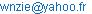(W. Nzié)

Josué Dzudié Fonsso, Wolfgang Nzié, Guy Edgar Ntamack, Bienvenu Kenmeugné. Hertz Theory Application in Modeling and Analysis of Mechanical Rupture Force of Cocoa Pod. International Journal of Mechanical Engineering and Applications. Vol. 4, No. 5, 2016, pp. 182-188. doi: 10.11648/j.ijmea.20160405.13

Received: August 11, 2016; Accepted: August 27, 2016; Published: September 21, 2016

Abstract: Cocoa seeds damage is a major problem facing cocoa farmers during breaking process. Hertz's theory on the contact forces was used for prediction of the rupture force just required to break a cocoa pod under compressive load along its lateral axis. Different varieties of cocoa pods were first subjected to compressive forces. Then they were placed on a test bench and subjected to the impact forces of steel mass dropped at different heights. The objective of this study is to improve the productivity of cacao pods breaker predicting the necessary rupture force. The model of the rupture force obtained from Hertz's theory performed good results compared with experimental values measured in the process of breaking the pods. The average ruptures forces of the cacao pods varieties "Criollo", "Forastero" and "Trinitario" have been determined. Rigidity (stiffness) of the pod was obtained from the force-deformation curve. There is a strongest correlation between the pod rigidity and the rupture force.

Keywords: Modeling, Rupture Force, Hertz Theory, Cocoa Pods, Stiffness Modulus, Force-Deformation Curve

1. Introduction

Cocoa is produced in Central and West Africa (particularly in Cameroon, in Latina America and Asia. Cocoa pod is a composite material constituted of external part, the shell, the placenta and seeds. The principal use and economic importance of cocoa are in medicine and production of chocolate, oil, petrol, candles, etc. . Cocoa farmers face problems of productivity that contribute to the reduction of their profit margins because of tools and technical methods used in different steps of valorization of this agricultural product. The breaking pod process is the first one after the harvest. Nowadays in many production zones this process is done manually with wood or machete; and is dangerous, non-productive because done on each cocoa pod one by one, and sometimes cocoa seeds are destroyed or cut. Indeed the rupture force is not well assessed. The modeling of this force is then relevant for cocoa pod breakers designers. A quantitative analysis of cocoa pod behavior should permit to identify and determine mechanical parameters, and in particular the minimal rupture force.

It has been confirmed that breaking process of cocoa pod aims to extract cocoa seeds from placenta. And the force needed for that purpose should be compressive, shearing or impact one according to machine design technology and process [2-4]. Compression testing of cocoa pods gives objective method to determine mechanical proprieties that permit to assess the minimal allowable load to break cocoa pods without destroying seeds. Although agricultural materials are generally non homogeneous, non-isotropic and non-elastic one can define a range of elastic behaviors in which parameters can be evaluated. In small deformations many agricultural materials are elastic and Hertz theory of contact forces can be applied . Generally biological materials can be breaking on compression load following force-strain straight curve. This model has been observed on soybeans, corncob, cocoa pods, macadamia nuts, rough rice and pea seeds [6-11].

Besides one can assume that errors resulting in the application of Hertz analysis for small deformations when breaking cocoa pods would be minimal considering only the elastic zone of force-deformation behavior. And also the rupture ends just after elastic limit. For fragile materials rupture can occur in the first part of force-deformation curve before the elastic limit, whereas it can happen considerably after the plastic zone of hard materials . Adopting methods used by [12-13] in their breaking studies of eggs, the rupture force and the maximal deformation had been measured to define the rigidity modulus. In this work, firstly cocoa pods will be broken along their lateral axis where its rupture force is to be low in compression according to studies on mechanical proprieties of similar materials . Then the rupture force analysis based on Hertz theory of cocoa pods will be performed assuming they are subjected to lateral compression between two steel plates. Finally the objectives of this study are the following:

To adapt the Hertz theory of contact stresses to predict the rupture force and compare it to one determined by experimental methods used, to afford best theory in the understanding of breaking process of cocoa pods to breakers designers;

To determine mechanical characteristics of different cocoa pods varieties.

2. Theoretical Development

2.1. Fundamental Hertz Relationships

They were developed for two bodies in contact under a load applied in static compression considering following assumptions: (1) the material is homogeneous; (2) contact stress is over a small area relative to the material size; (3) radii of curvature of the contracting surfaces are substantially greater than radius of the contact surface; (4) the surfaces are smooth .

The maximum stress occurs at the center of the contact area on the axis of the load where both bodies come into contact first. It is given by formula (1) [14-16].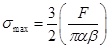(1)

F is the load, α and β are major and minor axis of the contact area which is elliptical.

2.1.1. Apparent Module of Elasticity

The apparent elasticity modulus of bodies of convex shape can be determined using Hertz equations theory. The combined deformation (D) in meters of two bodies along the load axis at the contact point is expressed according to :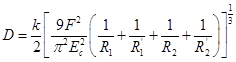(2)

Ec is the contact modulus given by formula (3):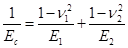(3)

E1 and E2 elasticity moduli of the compressed and compressing bodies respectively, in Nmm-2;

ν1, ν2 are poisson coefficients of precedent bodies respectively.

The major and minor axes in mm of the contact area which is elliptical are expressed by: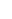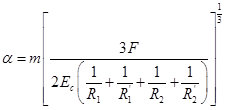(4)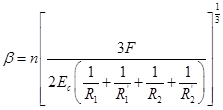(5)

k, m, n: Factors depending on the principal curvature of the body at the contact area and the angle θ between the normal planes containing the principal curvatures. The values of k,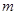and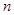are available in literature for various different angles θ , .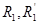: Minimum and maximum radii of curvature of compressed body (mm);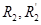: Minimum and maximum radii of compressing body (mm);

The manual breaking of cacao pod between two stones can be approximated to the compression of a spherical object between two rigid parallel plates, or the breaking of a cocoa pod impacting the wall of a pod breaker. So the following assumptions can be valid:

(a).  For the spherical compressed body (Cocoa pod) with geometric mean diameter dg in mm,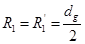;

(b).  For the compressing body (flat plate),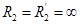, thus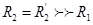;

(c).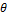= 90°, m = n = 1, k = 1.3514.

The compressing tool is a rigid plate of aluminum alloy or a cylindrical steel plate. Its E2 modulus is greater than cocoa pod modulus E1 which can then be expressed from formula (3) which second term is almost zero, thus formula (6):(6)

Using the conditions (a), (b), (c) in (2), the total deformation D of the solid (cocoa pod) at contact center becomes: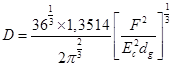(7)

Substituting (6) into (7) we get the following expression for the elasticity modulus of the cocoa pod.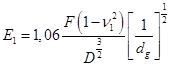(8)

2.1.2. Application of Hertz Theory Equation for Cocoa Pod Breaking

Adapting Hertz theory within applying a normal load F on a cocoa pod placed horizontally between two steel plates till rupture, it becomes maximal, and is function of normal displacement (D), geometric mean diameter (dg), Modulus of elasticity (E1) and poisson coefficient ν1 from equation (8). Thus the rupture force of cocoa pod is: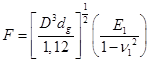(9)

The stiffness modulus S characterizes the slope of the force-deformation curve in the elastic region measured in N.mm-1. A pod under compression behaves as an elastic material.

Toughness in MJ.m-3 is defined as the ability of a material to absorb energy before its rupture. This can be approximated by the area under force-deformation curve up to the point of rupture [18-20].

Assuming that the variation of the contact area A0 is proportional to the variation of the applied force F (Hook law), based on Hertz theory, the stiffness modulus S of cocoa pod can be valuated as follows: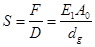(10)

From the stiffness modulus, the apparent modulus of elasticity (E1) is obtained from the equation (10):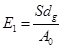(11)

Equation (12) is obtained by substituting equation (11) into equation (9):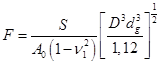(12)

2.2. Modification of Hertz Theory Equation

The sphericity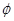is multiplied by the geometric mean diameter dg of cocoa pod to obtain expression of the apparent diameter da which has been considered in this analysis.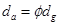(13)

The modified Hertz theory equation of cocoa pod rupture force has been expressed substituting dg by da in the equation (14):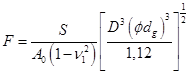(14)

3. Materials and Experimental Methods

3.1. Samples Preparation

Samples of ripened cocoa pods of three varieties grown in Cameroon: "Criollo", "Forastero" and "Trinitario" were harvested. For each variety shown in Figure 1, different sizes of ripened cocoa pods were selected for the study.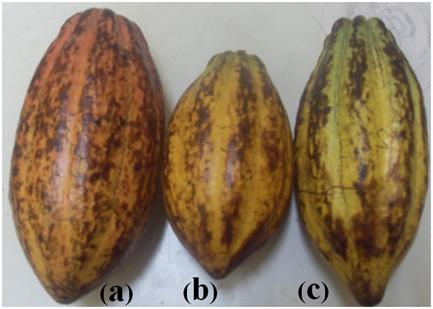Figure 1. Different varieties of cocoa pods: (a) Criollo, (b) Forastero and (c) Trinitario.

3.2. Test Equipment and Methodology

3.2.1. Physical Characteristics

Physical properties considered in this study are size and shape. For each cocoa pod three linear dimensions were measured, that is length (a), intermediate diameter (b) and major diameter (c), using a vernier caliper (ROCH-France) reading to 0.02 mm.

The geometric mean diameter (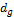) was determined  from the main principal physical dimensions.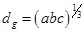(15)

Expression of sphericityof the pod was defined, according to , as: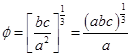(16)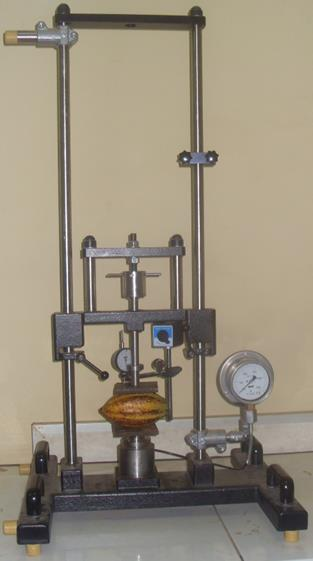Figure 2. Experimental device universal testing GUNT HAMBURG (model 198079) used for compressibility.

3.2.2. Uniaxial Compression Test Between Two Rigid Plates

Samples of the three cocoa varieties were subjected to uniaxial compression tests. Each pod has been compressed between two parallel steel plates using a universal testing machine GUNT HAMBURG (model 198079) as shown in Figure 2. The compression force was applied progressively until the pod completely broke. The experiments were repeated several times on the pods of different sizes and varieties. The test force was measured using a hydraulic force measuring device. Immediately after the breaking of pod, the average moisture content of the husks has been obtained using the oven method . Each of the different varieties has been placed in oven at a temperature of 105°C for 24 hours.

For determination of the contact surface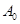it was possible to obtain the mesh of the footprint of the actual contact area between the compression tool and the pod under uniaxial compression from an impression stained on sheets of white paper placed in-between them.

3.2.3. Drop-Weight Experiment

The different varieties of cocoa pods were drawn from the same lot for which the physical properties were determined.

A test rig was set up to drop a solid mass of 2.5 Kg from different heights to break pods one by one. The different heights from which pods were broken were recorded. Ryder has shown that, for a load W in Newton (N), dropping at a certain height h in meters (m) onto a body with a material stiffness S in Newton/meter (Nm-1), the equivalent impact force F in N is given by the expression :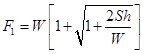(17)

4. Results and Discussion

4.1. Physical Properties

Average values of pod physical dimensions of cocoa varieties selected are given in Table 1. The cocoa pods dimensions were not significantly different (P ≤ 0.05) for all three varieties at given humidity.

The average values of the geometric mean diameter of the pods were 108.37 mm ± 8.99, 106.90 mm ± 8.59 and 105.89 mm ± 5.42 for "Criollo", "Forastero" and "Trinitario", respectively. The external and internal (furrow) thickness averages of the husks that fall in the range of physical dimensions were evaluated from multiple thickness measurements. Statistical analysis (Table 1) gave an overall average of the sphericity of 0.68 and also showed no significant difference with the different varieties of pods.

The pods deviate from the spherical shape with an average sphericity of 0.66 ± 0.08 mm for "Criollo" 0.70 ± 0.05 mm for "Forastero" and 0.67 ± 0.07 mm for "Trinitario".

Table 1. Some physical characteristics of cocoa pod of varieties.

 Physical properties Variety of the cocoa pod Criollo Forastero Trinitario Mean value Min. value Max. value Mean value Min. value Max. value Mean value Min. value Max. value Dimensions, mm Length, a 167.14±34.06 134.40 248.10 154.25±20.85 129.70 192.60 159.81±22.17 132.20 196.70 Intermediate diameter, b 86.15±6.03 76.30 94.40 87.49±5.67 75.70 100.30 84.36±3.30 78.40 90.15 Equatorial diameter, c 89.46±6.37 78.70 97.90 90.88±6.61 77.00 108.30 88.70±3.97 81.80 95.50 Geometric mean diameter, dg 108.37±8.99 95.60 129.22 106.90±8.59 91.10 127.90 105.89 ±5.42 96.59 113.41 Sphericity, ɸ 0.66±0.08 0.52 0.80 0.70±0.05 0.63 0.80 0,67±0.07 0.58 0.79 Apparent diameter, da 71,39±7.79 61,08 85.03 74.47±5,71 63.98 84.93 70.80±5.30 64.81 82.46 External thickness 12.96±1.05 11.40 14.30 14.58±0.88 13.30 16.30 13.63±1.81 11.00 16.80 Internal thickness (furrow) 11.31±0.77 10.10 12.40 13.03±0.79 11.90 14.20 11.32±1.48 9.90 14.30 Mass of the cocoa pod, g 482.1±99.7 338.2 686.2 520.6±113.1 340.4 797.5 564.6±116.9 437.6 781.4

4.2. Force-Deformation Behavior

When applying progressively a non-destructive load on the pod the behavior of the curve shows the dependence of the force according to linear displacement. Results of compressing different varieties of cocoa pods under a load between two parallel plates at a constant compression rate of loading are shown in Figure 3. The moisture content of the shells after the compression was 81.74% for "Criollo" and "Forastero", 82.74% for "Trinitario"

Under compressive loading along the lateral axis, deformation is elastic until the maximum breaking load. Damage of the pod is characterized by a macroscopic crack which extends from the load point. When the crack reaches the interface between the husk and the internal void, the pod explodes and there is a sudden loss of strength and a very slight increase in the maximum displacement.

The force required to break the pod internal void varies between 480 N to 680 N, 580 N to 820 N and 520 N to 650 N respectively for "Criollo", "Forastero" and "Trinitario". The Forasteto variety offers greater resistance to breaking. This must be due to the thickness of the shell. The general nature of these force-deformation curves in the lateral axis is similar to those of agricultural products such as Australia hazelnuts , various karingda seeds , sunflower seeds , and wheat kernels .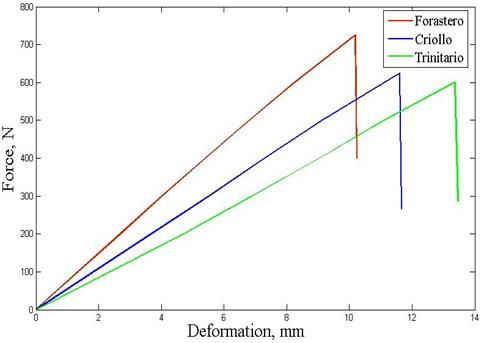Figure 3. Force-deformation curve of the three varieties of cocoa under compression along the lateral axis (plotted values are averages of samples).

The force-deformation curve obtained was analyzed to obtain the rigidity of the pod module. The energy absorbed (Ea) by a pod at the moment of rupture is determined by calculating the area under the force-deformation curve from the following expression: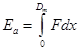(18)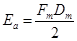(19)

Dm is the displacement (deformation) of the pod when the crack starts in compression. The extent of the cracking can be characterized by an energy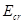: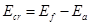Or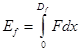(20)

Df is the final displacement at the end of crack. The rigidity modulus is regarded as the ratio of the breaking force and the displacement by compression. For each cocoa pod, the stiffness modulus was obtained from equation (11).

The average values for mechanical parameters describing the rupture of cocoa pods are given in Table 2. The dependence of the rupture force and the stiffness modulus of each variety is shown in Figure 4 (a), (b) and (c). The force and stiffness modulus of all varieties "Criollo", "Forastero" and "Trinitario" are positively correlated linearly.

Table 2. Experimental test results in lateral compression of cocoa varieties.

 Mechanical Parameters Varieties Fr (N) D (mm) S (N/mm) Ea (Nmm) Ef (Nmm) Ecr = Ea - Ef Criollo 625 11.6 54.79 2488.24 3597.08 1108.84 Forastero 720 10.18 71.58 2505.89 3640.22 1134.33 Trinitario 600 13.36 45.17 2411.76 3 398.13 986.37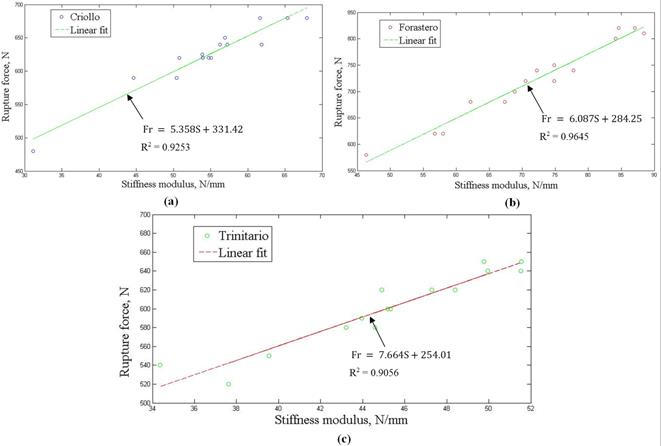Figure 4. Cocoa pod rupture force vs. stiffness modulus: (a) Criollo, (b) Forastero, (c) Trinitario.

4.3. Verification of the Hertzian Model

Values based on the model obtained by applying the Hertz theory and those obtained experimentally by methods used are shown in Table 3.

The slope of the linear portion of the curve force-deformation, which indicates material stiffness S, gave values of 54.34 N.mm-1, 72.36 N.mm-1 and 44.58 N.mm-1 for "Criollo", "Forastero" and "Trinitario" varieties, respectively. These values are used in the model obtained from the Hertz's equations. The predicted breaking force, based on Hertz’s theory, was compared with the values obtained during experimental testing using the two tests. The values obtained from the lateral compression though higher are not significantly different (P <0.05) with Hertz model values obtained. However those obtained experimentally during the drop-weight are higher and significantly different to the values obtained from the model and also the values obtained in compression tests. Thus the observations in this respect are in agreement with the employed experimental method (compression tests) which shows a good prediction of the model proposed from the Hertz theory. From the model and experimental findings, the trend is that fracture load increases with coco pod diameter. The same trend in the fracture of macadamia nuts is reported in .

Table 3. Comparison of the breaking force predicted and measured in cracking cocoa pods.

 Varieties physical parameters Rupture Force, N Geometric mean diameter, mm Apparent diameter (mm) Equivalent contact area, Ao (mm2) Hertzian model Lateral compression Falling weight impact Criollo 108.37 71.39 2205.60 609.66 625 1288.70 Forastero 106.90 74.47 2117.82 740.55 720 1600.11 Trinitario 105.89 70.80 2668.77 504.59 600 1120.87

5. Conclusion

Compressive strength properties of the cocoa pod were determined from the characteristics of the force-deformation curve. Hertz’s theory of contact stresses was applied to model and assess the rupture force of cocoa pods in uniaxial compression between two parallel plates along the lateral axis of the pod. It depends on the stiffness of the pod and was determined from the slope describing the behavior of the force-deformation curve in the elastic region. Using a theoretical expression derived from Hertz theory model for the process of cacao pod breaking, the minimum force required was also determined among many other mechanical materials parameters. The prediction of rupture force obtained from Hertz theory equation for cocoa pods is almost in agreement with experimental results obtained during compression tests of cocoa pods between two rigid parallel plates. The perspective of this work shall investigate Ryder formula (17) that gives almost double rupture force compared with the previous.

References

1. L. K Opeke,"Tropical Tree Crops",JohnandSons,Clichester,pp.108-119, 1987.
2. I. Audu, A. O. Oloso, B. Umar, "Development of a concentric cylinder locust dehullerˮ CIGR-Ejournal, PM 04 003 Vol. 6, 2004.
3. A. Vejesit, V. Salokhe, "Studies on machine-crop parameters of an axial flow thresher for threshing soy bean". CIGR-Ejournal Vol. 6, July, 2004
4. B. A. Adewumi, A. Fatusin, "Design, Fabrication and Testing of an Impact-Type Hand Operated Cocoa Pod Breaker,ˮ Agricultural Engineering International: the CIGR Ejournal, Manuscript PM 06 022 Vol. 8, 2006.
5. N. N. Mohsenin, "Physical properties of plant and animal materials", Gordon and Breach Science Publishers, New York, 1985.
6. M. R. Paulsen, "Fracture resistance of soybeans to compressive loading," Transactions of the American Society of Agricultural Engineers, 21(8), pp. 1210–1216, 1978.
7. U. G. N. Anazodo,  "Mechanical properties of the corncob under quasi-static radial compression,ˮ, Transactions of the ASAE, 26(4), pp. 1222–1228, 1983.
8. J. N. Maduako, M. O. Faborode, "Characterization of the breaking behaviour of whole cocoa pods" Journal of Agricultural Engineering Research, 59, pp. 89–96, 1994.
9. C. H. Wang, Y.W. Mai, "Deformation and fracture of Macadamia nuts. Part 2: microstructure and fracture mechanics analysis of nutshell," International Journal of Fracture, 69, pp. 67–85, 1994.
10. D. Shitanda, Y. Nishiyama, S. Koide, "Compressive strength properties of rough rice considering variation of contact area," Journal of Food Engineering, 53, pp. 53–8, 2002.
11. G. Lysiak, "Fracture toughness of pea: Weibull analysis," Journal of Food Engineering 83, pp. 436–43, 2007.
12. G. E. Rehkugler,"Egg handling equipment design" Transaction American Society of Agricultural Engineers, 7(2), pp. 174-187, 1964.
13. F. N. Reece, B. D. Lot, "The effect of loading rate on the breaking force, deformation and stiffness modulus of eggs," Poultry Science, 55, pp. 349–358, 1976.
14. F. B. Seely, J. O. Smith, "Advanced mechanics of materials", New York: John Wiley and Sons, 1965.
15. S. P. Timoshenko, J. N. Goodier, "Theory of elasticity", 3rd ed. New York: McGraw-Hill, 1970.
16. J. E. Shigley, C. R. Mishke, "Mechanical engineering design". 6th ed. NewYork: McGraw-Hill, 2001.
17. ASAE, "Compression test of food materials of convex shape", ASAE (American Society of Agricultural Engineers) Standards, S368.3. St. Joseph, Michigan, 1998.
18. M. O. Faborode, R. R. Dirinfo, "A mathematical model of cocoa pod deformation based on Hertz theory," International Agrophysics 8, pp. 403–409, 1994.
19. M. Grotte, F. Duprat, D. Loonis, "Mechanical Properties of the Skin and the Flesh of Apples," International Journal of Food Properties, 4(1), pp. 149–161, 2001
20. R. K. Gupta, S. K. Das, "Fracture resistance of sunﬂower seed and kernel to compressive loading," Journal of Food Engineering, 46(1), pp. 1–8, 2000.
21. R. K. Jain, S. Bal, "Physical properties of pearl millet," Journal of Agricultural Engineering Research, 66, pp. 85–91, 1997.
22. S. H. Suthar, S.K. Das, "Fracture resistance of Karingda (Citrullus lanatus (Thumb) Mansf) seeds to compressive loading," Journal of Food Engineering, 34, pp. 77–90, 1997.
23. Sayyah Aha, S. Minaei, "Behavior of wheat kernels under quasi-static loading and its relation to grain hardness," Journal of Agricultural Sciences and Technology, 6, pp. 11–19, 2004.

 Contents 1. 2. 2.1. 2.2. 3. 3.1. 3.2. 4. 4.1. 4.2. 4.3. 5.
Article ToolsAbstractPDF(1065K)